Next: Absorption and Stimulated Emission Up: Time-Dependent Perturbation Theory Previous: Energy-Shifts and Decay-Widths

# Harmonic Perturbations

Consider a perturbation to the Hamiltonian that oscillates sinusoidally in time. This is usually termed a harmonic perturbation. Thus,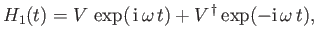(8.110)

whereis, in general, a function of the position, momentum, and spin operators.

Let us initiate the system in the eigenstate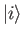of the unperturbed Hamiltonian,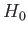, and then switch on the harmonic perturbation at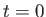. It follows from Equation (8.59) that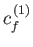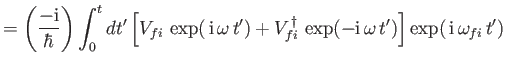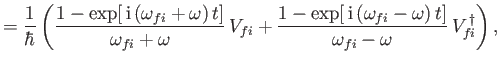(8.111)

where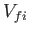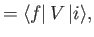(8.112)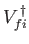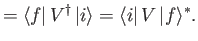(8.113)

Equation (8.114) is analogous to Equation (8.66), provided that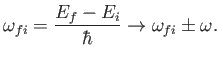(8.114)

Thus, it follows from the analysis of Section 8.6 that the transition probability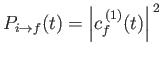is only appreciable in the limit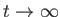if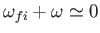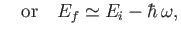(8.115)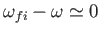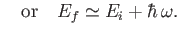(8.116)

Clearly, criterion (8.118) corresponds to the first term on the right-hand side of Equation (8.114), whereas criterion (8.119) corresponds to the second. The former term describes a process by which the system gives up energy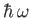to the perturbing field, while making a transition to a final state whose energy is less than that of the initial state by. This process is known as stimulated emission. The latter term describes a process by which the system gains energyfrom the perturbing field, while making a transition to a final state whose energy exceeds that of the initial state by. This process is known as absorption. In both cases, the total energy (i.e., that of the system plus the perturbing field) is conserved.

By analogy with Equation (8.79),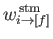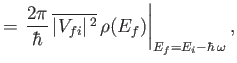(8.117)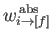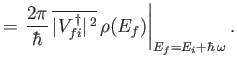(8.118)

Equation (8.120) specifies the transition rate for stimulated emission, whereas Equation (8.121) gives the transition rate for absorption. These transition rates are more usually written [see Equation (8.80)]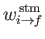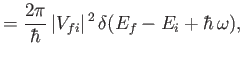(8.119)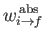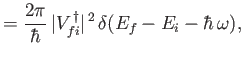(8.120)

where it is understood that the previous expressions must be integrated with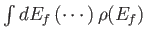to obtain the actual transition rates.

It is clear from Equations (8.115) and (8.116) that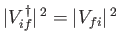. It follows from Equations (8.120) and (8.121) that(8.121)

In other words, the rate of stimulated emission, divided by the density of final states for stimulated emission, is equal to the rate of absorption, divided by the density of final states for absorption. This result, which expresses a fundamental symmetry between absorption and stimulated emission, is known as detailed balance, and plays an important role in quantum statistical mechanics.Next: Absorption and Stimulated Emission Up: Time-Dependent Perturbation Theory Previous: Energy-Shifts and Decay-Widths
Richard Fitzpatrick 2016-01-22